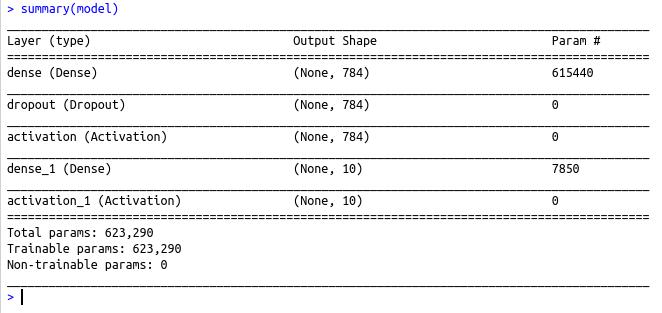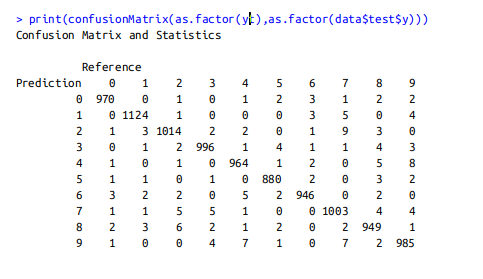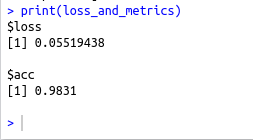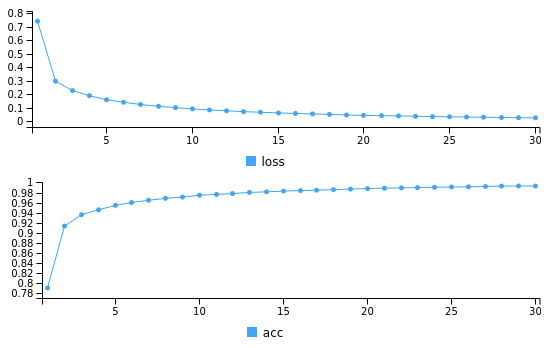• #5, First Floor, 4th Street Dr. Subbarayan Nagar Kodambakkam, Chennai-600 024 Landmark : Samiyar Madam
• pro@slogix.in
• +91- 81240 01111

#### Social List

##### How to classify handwritten characters using deep neural network in R?
###### Description

To classify handwritten characters using deep neural network in R

#### Functions Used

model layer_dense() – Add a input,output or hidden layer
layer_activation() – Activation function for the layer
compile() – To compile the model
fit() – To fit the model using the train set
predict() – To predict using the test set
evaluate() – To evaluate the loss and metrics

#### Libraries Required :

library(keras)

###### Process

Convert the categorical variables to equivalent numeric classes

Split the data set as train set and test set

Initialize the keras sequential model

Build the model with input layers,hidden layers and output layer as per the data sizealong with the activation function(here 748 I/P layer with relu activation and 10 O/P layer with softmax activation)

Compile the model with required loss,metrics and optimizer(here loss=categorical_crossentropy,optimizer=adam,metrics=accuracy)

Fit the model using the train set

Predict using the test set

Evaluate the metrics

###### Sapmle Code

library(keras)
library(caret)
data<-dataset_mnist()
#separating train and test file
train_x<-data\$train\$x
train_y<-data\$train\$y
test_x<-data\$test\$x
test_y<-data\$test\$y
# converting a 2D array into a 1D array for feeding into the MLP and normalising the maProcess :

Convert the categorical variables to equivalent numeric classes
Split the data set as train set and test set
Initialize the keras sequential model
Build the model with input layers,hidden layers and output layer as per the data size along with the activation function(here 748 I/P layer with relu activation and 2 O/P layer with softmax activation)
Compile the model with required loss,metrics and optimizer(here loss=binary_crossentropy,optimizer=adam,metrics=accuracy)
Fit the model using the train set
Predict using the test set
Evaluate the metrics
trix
train_x1 test_x1 #converting the target variable to once hot encoded vectors using keras inbuilt function
train_y<-to_categorical(train_y,10)
test_y<-to_categorical(test_y,10)
#defining a keras sequential model
model %
layer_dense(units = 784, input_shape = 784) %>%
layer_dropout(rate=0.4)%>%
layer_activation(activation = ‘relu’) %>%
layer_dense(units = 10) %>%
layer_activation(activation = ‘softmax’)

#compiling the defined model with metric = accuracy and optimiser as adam.
model %>% compile(
loss = ‘categorical_crossentropy’,
metrics = c(‘accuracy’)
)

#Summary of the model
summary(model)
#fitting the model on the training dataset
model %>% keras::fit(train_x1, train_y, epochs = 30, batch_size = 2000)

#Evaluating model on the cross validation dataset
loss_and_metrics % evaluate(test_x1, test_y, batch_size = 2000)
print(loss_and_metrics)
#Predict using the Test data
yt=predict_classes(model,test_x1)
cat(“The confusion matrix is \n”)
print(confusionMatrix(as.factor(yt),as.factor(data\$test\$y)))
##Color ramp
colors<-c(‘white’,’black’)
cus_col<-colorRampPalette(colors=colors)
## Plot the average image of each digit
par(mfrow=c(4,3),pty=’s’,mar=c(1,1,2,1),xaxt=’n’,yaxt=’n’)
for(di in 1:12)
{
image(1:28,1:28,t(test_x[di,,])[,28:1],main=paste(“Predicted : “,yt[di]),col=cus_col(256))
}

###### Screenshots